# Chapter 2 : Measure of Central Tendency

### Topics covered in this snack-sized chapter:

#### Measure of Central Tendency arrow_upward

• A measure of central tendency is a single value that attempts to describe a set of data by identifying the central position within that set of data.
•  It can be measured by:
• Mean (Arithmetic Mean)
• Median
• Mode
• The Geometric Mean
• Variation can be measured by:
• Range
• Quartile
• Variance
• Standard Deviation
• Coefficient of Variation

#### Mean arrow_upward

• It is the most common measure of central tendency.
• The mean is equal to the sum of all the values in the data set divided by the number of values in the data set.
•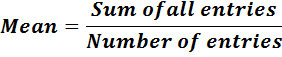• Example: Find the mean of the following:
• {66, 72, 83, 89}
• Solution:
• Mean = (66 + 72 + 83 + 89) / 4
• => Mean = 310 / 4
• => Mean = 77.5

###### Sample Mean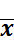:

• If n is the sample size then we have:
•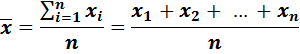• Where,
• Sum of all values =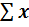• If the data represents a sample, the number of entries = n

###### Population Mean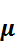:

• If N is population size then we have:
•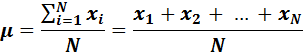• Where,
•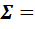Sum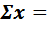Sum of all values of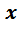• If the data represents an entire population, the number of entries =N

#### Mean from a Frequency Distribution arrow_upward

• It is used when the only source of data is a frequency distribution.
•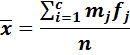• Where,
• n = Sample size,

c = Number of classes in the frequency distribution,

mj = Midpoint of the jth class,

fj = Frequency of the jth class

#### Median arrow_upward

• The median is the middle score for a set of data that has been arranged in ascending or descending order.

##### Step: 1
• Arrange numbers in ascending order.
• ##### Step: 2A
• For odd numbers:
•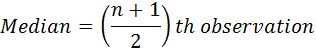##### Step: 2B
• For even numbers median is the average of the (n/2)th observation and the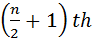observation.
• Example 1: Find the median of the following:
• 72, 65, 81, 89, 83
• Solution: Arrange the numbers in ascending order.

65, 72, 81, 83, 89

• For odd numbers:
•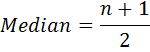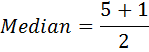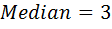• Here the median is 3rd observation that is 81.
• Example 2: Find the median of the following:
• {31, 57, 12, 22, 43, 50}
• Solution: Arrange the numbers in ascending order.
• 12, 22, 31, 43, 50, 57
• For even numbers:
• The median is the average of the middle two values,
•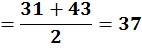• Here median is 37.

• #### Outliers arrow_upward

• Outliers are numbers in a data set that are either way bigger or way smaller than the other numbers in a data set.
• Example: In 1, 2, 3, 4, 4, 6 and 31 data set the number 31 is the outlier.

• ###### Mean is impacted by Outliers:

• If the outlier is a high value, it will cause the mean value to shift to the higher side, while a low valued outlier will drop the mean value to a lower number.
• Below example is showing how the mean is impacted by outliers:
•###### Median is not impacted by Outliers:

• Median is not impacted by outliers as shown below:
•#### Mode arrow_upward

• Mode is a number that occurs most frequently in the data set.
• Steps to find Mode:

• Step: 1
• Arrange the numbers in ascending or descending order.
• Step: 2
• Find the number which is occurring maximum number of times in the set.
##### Note:
• If there are two such numbers which occur maximum number of times then there is NO mode.
• Example 1: Find the mode of the following:
• {9, 3, 3, 7, 8, 15, 3, 9}

• Solution: Arrange the numbers in ascending order:
• 3, 3, 3, 7, 8, 9, 9, 15
• As 3 is occurring maximum number of times, 3 is the mode.
• Example 2: For data:
• 6, 7, 2, 5, 3, 4, 9, 8

• As no item in the data set given above is repeating itself so there will be No mode.

#### Range arrow_upward

• The difference between the largest and the smallest values of a distribution is known as range.
• Example 1: The range of 10, 13, 17, 17, 18 will be:
•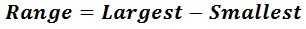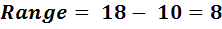#### Percentiles arrow_upward

• Percentile is the value of a variable below which a certain percent of observations fall.
• Example: The 30th percentile is the value below which 30 percent of the observations may be found.
• The percent falling above the percentile Pth will be (100 – P)%.
•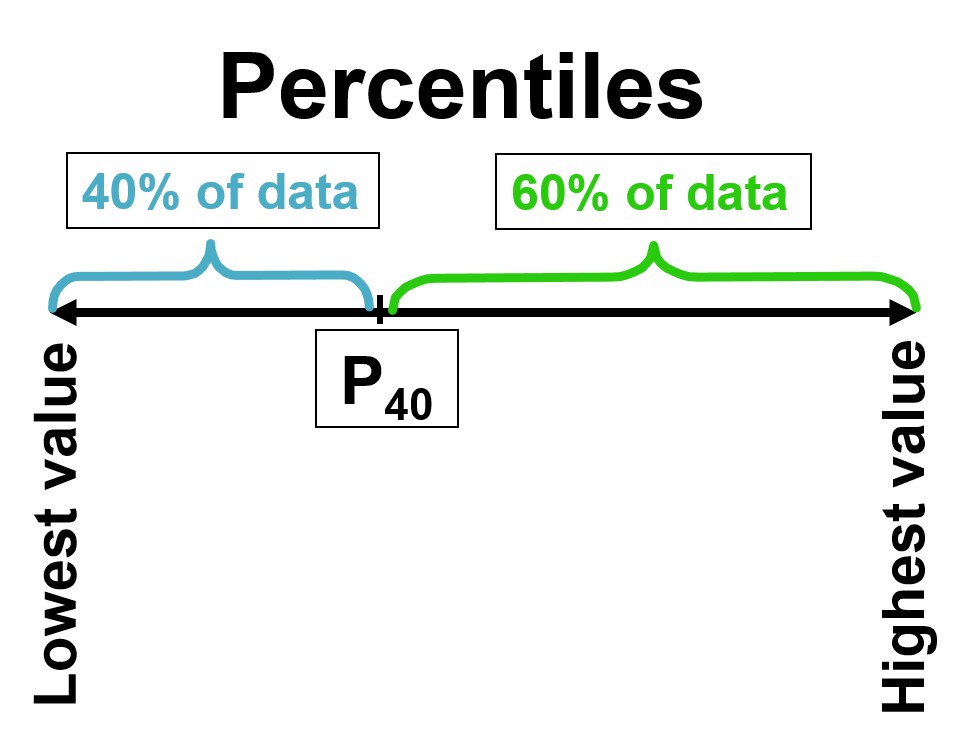#### Quartiles arrow_upward

• Quartiles split ordered data into 4 equal portions.
• Each Quartile has position and value.
•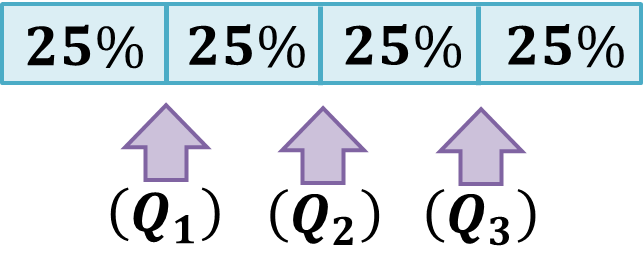• With the data in an ordered array, the position of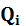is:
•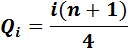• The value ofis the value associated with that position in the ordered array.

• #### Interquartile Range (IQR) arrow_upward

• It is also known as mid-spread or middle fifty.
• It is equal to the difference between upper quartile Q1 and lower quartile Q3 .
• The middle is 50% of the values.
• Resistant to extreme values.
• Interquartile range = IQR = Q3 - Q1
•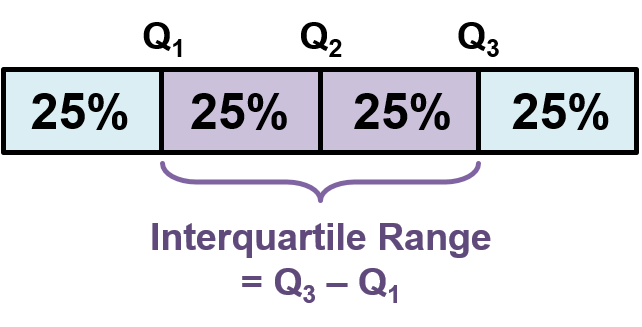#### Thank You from Kimavi arrow_upward

• Please email us at Admin@Kimavi.com and help us improve this tutorial.